# Cartesian-closed category

(diff) ← Older revision | Latest revision (diff) | Newer revision → (diff)

A category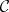such that the following axioms are satisfied:

A1) there exists a terminal object;

A2) for any pair,of objects ofthere exist a product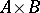and given projections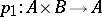,;

A3) for any pair,of objects ofthere exist an objectand an evaluation arrowsuch that for any arrowthere is a unique arrowwith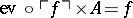.

These conditions are equivalent to the following:is a category with given products such that the functors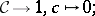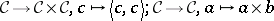have each a specified right-adjoint, written respectively as:Some examples of Cartesian-closed categories are:

E1) any Heyting algebra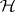;

E2) the category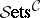for any small categorywith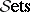the category of (small) sets — in particularitself;

E3) the category of sheaves over a topological space, and more generally a (Grothendieck) topos;

E4) any elementary topos;

E5) the category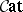of all (small) categories;

E6) the category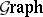of graphs and their homomorphisms;

E7) the category-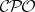of-CPOs.

These definitions can all be put into a purely equational form.

How to Cite This Entry:
Cartesian-closed category. Encyclopedia of Mathematics. URL: http://encyclopediaofmath.org/index.php?title=Cartesian-closed_category&oldid=14645
This article was adapted from an original article by M. Eytan (originator), which appeared in Encyclopedia of Mathematics - ISBN 1402006098. See original article